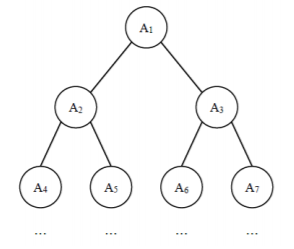# 完全二叉树的权值1≤N≤1051≤N≤105,
−105≤Ai≤105−105≤Ai≤105

7
1 6 5 4 3 2 1


2
import java.util.*;
public class Main{
public static void main(String[] args) {
Scanner sc = new Scanner(System.in);
int n = sc.nextInt();
long max = -100010;
int k = -1,ans = 0;
while(n > 0) {
int cnt = (int)Math.pow(2, ++ k);
long sum = 0;
for(int i = 1;i <= cnt && n > 0;i ++) {
sum += sc.nextInt();
n --;
}
if(sum > max) {
max = sum;
ans = k + 1;
}
}
System.out.println(ans);
}
}

©️2019 CSDN 皮肤主题: 大白 设计师: CSDN官方博客